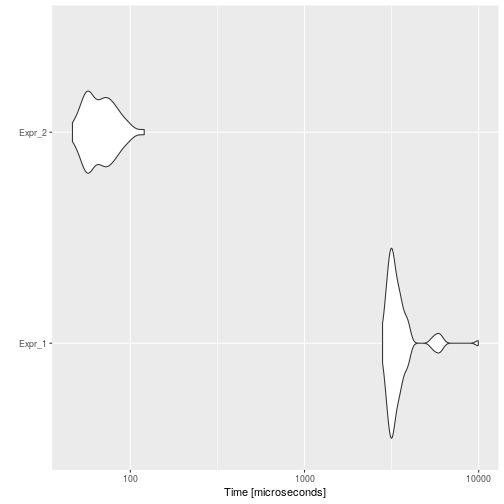# Loop-invariant Code Motion

## Background

Loop-invariant code consists of statements or expressions which can be moved outside the body of a loop without affecting the semantics of the program. Loop-invariant code motion is a compiler optimization which performs this movement automatically.

For example, consider the following code:

``````i <- 0
n <- 1000
y <- rnorm(1)
z <- rnorm(1)
a <- c()
while (i < n) {
x <- y + z
a[i] <- 6 * i + x * x
i <- i + 1
}
``````

Here, `x` is assigned a value `y + z` that is constant in each loop execution. In this example, the assignment would be executed `n` times. The same result, but much faster, would be obtained by doing the assign outside the loop.

## Example

Consider the previous example to be automatically optimized.

``````code <- paste(
"i <- 0",
"n <- 1000",
"y <- rnorm(1)",
"z <- rnorm(1)",
"a <- c()",
"while (i < n) {",
"  x <- y + z",
"  a[i] <- 6 * i + x * x",
"  i <- i + 1",
"}",
sep = "\n"
)
cat(code)
``````
``````## i <- 0
## n <- 1000
## y <- rnorm(1)
## z <- rnorm(1)
## a <- c()
## while (i < n) {
##   x <- y + z
##   a[i] <- 6 * i + x * x
##   i <- i + 1
## }
``````

Then, the automatically optimized code would be:

``````opt_code <- opt_loop_invariant(list(code))
cat(opt_code\$codes[])
``````
``````## i <- 0
## n <- 1000
## y <- rnorm(1)
## z <- rnorm(1)
## a <- c()
## if (i < n) {
##   x <- y + z
## }
## while (i < n) {
##   a[i] <- 6 * i + x * x
##   i <- i + 1
## }
``````

And if we measure the execution time of each one, and the speed-up:

``````bmark_res <- microbenchmark({
eval(parse(text = code))
}, {
eval(parse(text = opt_code))
})
autoplot(bmark_res)
````````````speed_up(bmark_res)
``````
``````##            Min.  1st Qu.   Median     Mean  3rd Qu.     Max.
## Expr_2 60.27293 54.23595 48.68204 51.43153 46.48122 82.67596
``````

## Implementation

The `opt_loop_invariant` optimizer does:

• Finds `for` and `while` loops in the code.

• Discards loops that have function calls inside, as function calls can edit the environment.

• Gets which variables are variant inside the loop.

• Detects which expressions do not use any variant variable.

• Gets these invariant variables, and moves them outside the loop, but inside an `if` statement (with the same conditional as the loop).

## To-Do

• `if/else` code motion?

Actually, the `opt_loop_invariant` does not consider `if/else` expressions to move. In this sense, the code:

``````while (i < n) {
if (n > 3) {
something_without_i
}
}
``````

Will not be optimized to its equivalent code:

``````if (i < n) {
if (n > 3) {
something_without_i
}
}
while (i < n) {
}
``````
• Invariant subexpressions motion?

Actually, the `opt_loop_invariant` considers only full expressions, it is not working on subexpressions, for instance, the code:

``````while (i < n) {
i <- (x * y) + 1
}
``````

as `x` and `y` are invariant, could be optimized to:

``````is_1 <- (x * y)
while (i < n) {
i <- is_1 + 1
}
``````
• Include `repeat` optimization?

Since determining the conditional that causes a `repeat` loop to stop is not that simple, it is not easy to remove invariant variables and place them in an `if`.

For example, the code:

``````y <- 1
repeat{
if (y > 4) {
break
}
x <- 8 * 8
y <- y + 1
}
``````

Must be optimized to:

``````y <- 1
if (y <= 4) {
x <- 8 * 8
}
repeat{
if (y > 4) {
break
}
y <- y + 1
}
``````